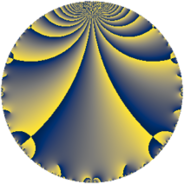# Properties

 Label 18.3.dLevel $18$ Weight $3$ Character orbit 18.d Rep. character $\chi_{18}(5,\cdot)$ Character field $\Q(\zeta_{6})$ Dimension $4$ Newform subspaces $1$ Sturm bound $9$ Trace bound $0$

# Related objects

## Defining parameters

 Level: $$N$$ $$=$$ $$18 = 2 \cdot 3^{2}$$ Weight: $$k$$ $$=$$ $$3$$ Character orbit: $$[\chi]$$ $$=$$ 18.d (of order $$6$$ and degree $$2$$) Character conductor: $$\operatorname{cond}(\chi)$$ $$=$$ $$9$$ Character field: $$\Q(\zeta_{6})$$ Newform subspaces: $$1$$ Sturm bound: $$9$$ Trace bound: $$0$$

## Dimensions

The following table gives the dimensions of various subspaces of $$M_{3}(18, [\chi])$$.

Total New Old
Modular forms 16 4 12
Cusp forms 8 4 4
Eisenstein series 8 0 8

## Trace form

 $$4 q + 4 q^{4} - 18 q^{5} - 12 q^{6} + 2 q^{7} + 12 q^{9} + O(q^{10})$$ $$4 q + 4 q^{4} - 18 q^{5} - 12 q^{6} + 2 q^{7} + 12 q^{9} + 18 q^{11} + 12 q^{12} - 10 q^{13} + 36 q^{14} + 18 q^{15} - 8 q^{16} - 24 q^{18} - 40 q^{19} - 36 q^{20} - 42 q^{21} - 12 q^{22} + 18 q^{23} + 4 q^{25} + 8 q^{28} + 18 q^{29} + 72 q^{30} + 38 q^{31} + 54 q^{33} + 24 q^{34} + 12 q^{36} + 128 q^{37} - 72 q^{38} - 102 q^{39} - 126 q^{41} - 48 q^{42} - 46 q^{43} - 54 q^{45} - 24 q^{46} + 54 q^{47} + 24 q^{48} - 12 q^{49} - 72 q^{51} + 20 q^{52} + 36 q^{54} - 108 q^{55} + 72 q^{56} + 144 q^{57} + 24 q^{58} + 126 q^{59} - 36 q^{60} + 62 q^{61} + 222 q^{63} - 32 q^{64} + 90 q^{65} - 72 q^{66} - 106 q^{67} + 72 q^{68} + 18 q^{69} - 108 q^{70} - 96 q^{72} - 208 q^{73} + 72 q^{74} - 12 q^{75} - 40 q^{76} - 90 q^{77} + 144 q^{78} + 14 q^{79} - 252 q^{81} + 144 q^{82} - 378 q^{83} - 144 q^{84} + 108 q^{85} - 108 q^{86} - 54 q^{87} + 24 q^{88} + 412 q^{91} + 36 q^{92} + 222 q^{93} + 84 q^{94} + 180 q^{95} + 48 q^{96} + 14 q^{97} + 126 q^{99} + O(q^{100})$$

## Decomposition of $$S_{3}^{\mathrm{new}}(18, [\chi])$$ into newform subspaces

Label Dim $A$ Field CM Traces $q$-expansion
$a_{2}$ $a_{3}$ $a_{5}$ $a_{7}$
18.3.d.a $4$ $0.490$ $$\Q(\sqrt{-2}, \sqrt{-3})$$ None $$0$$ $$0$$ $$-18$$ $$2$$ $$q+\beta _{1}q^{2}+(1-2\beta _{1}-2\beta _{2}+\beta _{3})q^{3}+\cdots$$

## Decomposition of $$S_{3}^{\mathrm{old}}(18, [\chi])$$ into lower level spaces

$$S_{3}^{\mathrm{old}}(18, [\chi]) \cong$$ $$S_{3}^{\mathrm{new}}(9, [\chi])$$$$^{\oplus 2}$$